#### A-star

The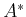(pronounced ay star'') search algorithm is an extension of Dijkstra's algorithm that tries to reduce the total number of states explored by incorporating a heuristic estimate of the cost to get to the goal from a given state. Let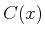denote the cost-to-come from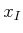to, and let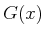denote the cost-to-go fromto some state in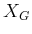. It is convenient that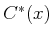can be computed incrementally by dynamic programming; however, there is no way to know the true optimal cost-to-go,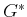, in advance. Fortunately, in many applications it is possible to construct a reasonable underestimate of this cost. As an example of a typical underestimate, consider planning in the labyrinth depicted in Figure 2.2. Suppose that the cost is the total number of steps in the plan. If one state has coordinates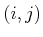and another has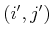, then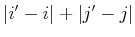is an underestimate because this is the length of a straightforward plan that ignores obstacles. Once obstacles are included, the cost can only increase as the robot tries to get around them (which may not even be possible). Of course, zero could also serve as an underestimate, but that would not provide any helpful information to the algorithm. The aim is to compute an estimate that is as close as possible to the optimal cost-to-go and is also guaranteed to be no greater. Let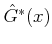denote such an estimate.

Thesearch algorithm works in exactly the same way as Dijkstra's algorithm. The only difference is the function used to sort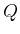. In thealgorithm, the sum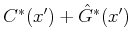is used, implying that the priority queue is sorted by estimates of the optimal cost fromto. Ifis an underestimate of the true optimal cost-to-go for all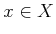, thealgorithm is guaranteed to find optimal plans [337,777]. As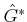becomes closer to, fewer vertices tend to be explored in comparison with Dijkstra's algorithm. This would always seem advantageous, but in some problems it is difficult or impossible to find a heuristic that is both efficient to evaluate and provides good search guidance. Note that when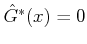for all, thendegenerates to Dijkstra's algorithm. In any case, the search will always be systematic.

Steven M LaValle 2020-08-14